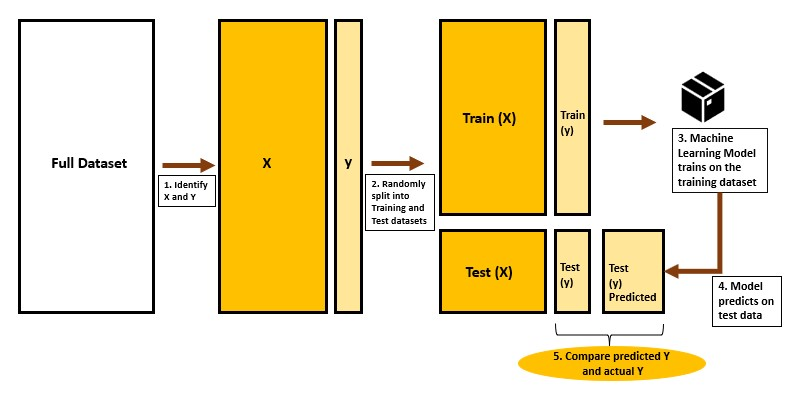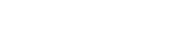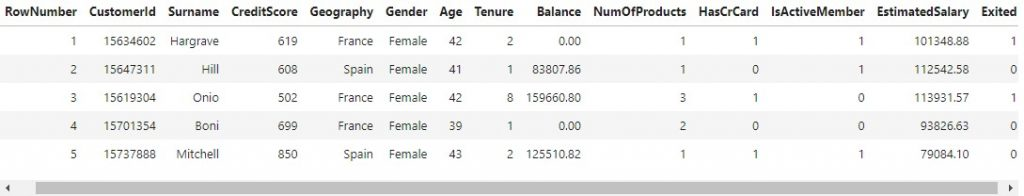# Train Test Split – How to split data into train and test for validating machine learning models?The train-test split technique is a way of evaluating the performance of machine learning models.

Whenever you build machine learning models, you will be training the model on a specific dataset (X and y). Once trained, you want to ensure the trained model is capable of performing well on the unseen test data as well. The train test split is a way of checking if the ML model performs well on data it has not seen.

This is applied to supervised learning problems, both classification and regression.

## How does the Train-Test split work?

So you have a dataset that contains the labels (y) and predictors (features X). Split the dataset randomly into two subsets:

1. Training set: Train the ML model
2. Testing set: Check how accurate the model performed.

On the first subset called the training set, you will train the machine learning algorithm and build the ML model.

Then, use this ML model on the other subset, called the Test set, to predict the labels. Then you can compare the labels thus predicted and the actual labels of the test set.

If the model is good, the label predictions will be close to the actual labels.## How to use Train Test split to check for overfitting?

A model that does not overfit will be able to predict the data it has not seen to the same level of accurateness as the data it has trained on.

How to actually check for overfitting?

Once you have trained the model on the training set, use the ML model and the features from BOTH the training and the test set to make label predictions.

Once you do this you will have both predicted values as well as actuals for both training and test sets.

Compare the performance of the model on both the training and test set, it should be fairly close. If there is a wide gap, that is, the performance on training is way better than the test, then the model to be overfitting.

## In what proportion to split the train and test datasets?

It is very common to split the train:test datasets in:

1. 70:30 ratio
2. 80:20 ratio
3. 75:25 ratio

There is no specific rule that you MUST split the data in this or that proportion. Only thing you need to consider is to make sure the ML model will have sufficient datapoints in the training data to learn from. If there is no shortage of datapoints, you can even split the train:test data in 50:50 ratio.

#### MLPlus Industry Data Scientist Program

Struggling to find a well structured path for Data Science?

Build your data science career with a globally recognised, industry-approved qualification. Solve projects with real company data and become a certified Data Scientist in less than 12 months and get Guaranteed Placement. .#### Get Free Complete Python Course

Build your data science career with a globally recognised, industry-approved qualification. Get the mindset, the confidence and the skills that make Data Scientist so valuable.

## Apply Train Test split

The train test split can be easily done using train_test_split() function in scikit-learn library.

from sklearn.model_selection import train_test_split


Import the data

import pandas as pd## Method 1: Train Test split the entire dataset

df_train, df_test = train_test_split(df, test_size=0.2, random_state=100)
print(df_train.shape, df_test.shape)

(8000, 14) (2000, 14)


The random_state is set to any specific value in order to replicate the same random split.

## Method 2: Train Test split X and y

# Prepare X and y
X = df.iloc[:,:-1]
y = df.iloc[:,-1]

# Do train test split
train_x, test_x, train_y, test_y = train_test_split(X, y, test_size=0.2, random_state=1)
print(train_x.shape, test_x.shape, train_y.shape, test_y.shape)

(8000, 13) (2000, 13) (8000,) (2000,)


Check the value counts. There can be small differences between the train and test because of randomization.

print("***Train y value counts***\n", train_y.value_counts(normalize=True))
print("***Test y value counts***\n", test_y.value_counts(normalize=True))

***Train y value counts***
0    0.79725
1    0.20275
Name: Exited, dtype: float64
***Test y value counts***
0    0.7925
1    0.2075
Name: Exited, dtype: float64


## Method 3: Stratified Train test split

When there is a class imbalance in Y, and you want to retain the same proportion of the individual classes of Y in the train-test splits, you can do stratified splits.

To do stratified splits, set the stratify parameter to the label column. Note, this does not accept ‘True’ or ‘False’.

# Prepare X and y
X = df.iloc[:,:-1]
y = df.iloc[:,-1]

# Do train test split
train_x, test_x, train_y, test_y = train_test_split(X, y, test_size=0.2, random_state=1, stratify=y)
print(train_x.shape, test_x.shape, train_y.shape, test_y.shape)

(8000, 13) (2000, 13) (8000,) (2000,)


Check the value counts, it will be the same because of stratified sampling.

print("***Train y value counts***\n", train_y.value_counts(normalize=True))
print("***Test y value counts***\n", test_y.value_counts(normalize=True))

***Train y value counts***
0    0.79625
1    0.20375
Name: Exited, dtype: float64
***Test y value counts***
0    0.7965
1    0.2035
Name: Exited, dtype: float64


## Similar Articles

### Logistic Regression – A Complete Tutorial With Examples in RCourse Preview

## Machine Learning A-Z™: Hands-On Python & R In Data Science

### Free Sample Videos:#### Machine Learning A-Z™: Hands-On Python & R In Data Science#### Machine Learning A-Z™: Hands-On Python & R In Data Science#### Machine Learning A-Z™: Hands-On Python & R In Data Science#### Machine Learning A-Z™: Hands-On Python & R In Data Science